# ISEE Middle Level Quantitative : Outcomes

## Example Questions

### Example Question #11 : How To Find The Probability Of An Outcome

Two boxes contain only red and green marbles. Box #1 contains five green marbles and ten red marbles; Box #2 contains ten green marbles and thirty red marbles. Which is the greater quantity?

(A) The probability that a marble randomly drawn from Box #1 is green

(B) The probability that a marble randomly drawn from Box #2 is green

(B) is greater

It is impossible to determine which is greater from the information given.

(A) is greater

(A) and (B) are equal

(A) is greater

Explanation:

Box #1 contains five green marbles and fifteen marbles overall; a marble randomly drawn from this box will be green with probability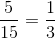Box #2 contains ten green marbles and forty marbles overall; a marble randomly drawn from this box will be green with probability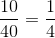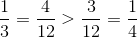so (A) is greater.

### Example Question #12 : How To Find The Probability Of An Outcome

Two boxes contain only red and green marbles. Box #1 contains eight green marbles and ten red marbles; Box #2 contains twenty green marbles and sixteen red marbles. Which is the greater quantity?

(A) The probability that a marble randomly drawn from Box #1 is green

(B) The probability that a marble randomly drawn from Box #2 is red

It is impossible to determine which is greater from the information given

(A) is greater

(A) and (B) are equal

(B) is greater

(A) and (B) are equal

Explanation:

Box #1 has eight green marbles out of eighteen marbles total; the probability that a randomly drawn marble is green is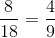.

Box #2 has sixteen red marbles out of thirty-six marbles total; the probability that a randomly drawn marble is green is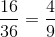.

The two probabilities are equal.

### Example Question #13 : How To Find The Probability Of An Outcome

Two standard decks of fifty-two cards, one with a yellow backing and one with a gray backing, are presented to you. The yellow deck has all of its aces removed; the gray deck is left complete. Which is the greater quantity?

(A) The probability that a card randomly drawn from the yellow deck is a six

(B) The probability that a card randomly drawn from the gray deck is a six

(B) is greater

(A) and (B) are equal

(A) is greater

It is impossible to determine which is greater from the information given

(A) is greater

Explanation:

The yellow deck has four sixes out of forty-eight cards; the probability of drawing a six at random is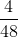.

The gray deck has four sixes out of fifty-two cards; the probability of drawing a six at random is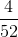.

Between two fractions with the same numerator, the fraction with the lesser denominator is the greater, so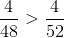. (A) is greater.

### Example Question #14 : How To Find The Probability Of An Outcome

A box contains fifteen blue marbles, ten green marbles, and twenty red marbles, and no other marbles. What are the odds against a marble randomly drawn from this box being blue?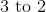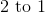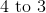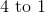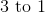Explanation:

Since there are 30 marbles that are not blue and 15 that are blue, this makes 30 unfavorable outcomes to 15 favorable outcomes. This makes the odds against this favorable outcome, in fraction form: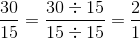or 2 to 1 odds.

### Example Question #15 : How To Find The Probability Of An Outcome

A box contains fifteen blue marbles, ten green marbles, twenty red marbles, and twenty-five white marbles, and no other marbles. What are the odds against a marble randomly drawn from this box being blue or green?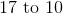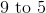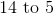Explanation:

There are 45 marbles that are neither blue nor green, and twenty-five marbles that are blue or green, so the odds against drawing a blue or green marble are 45 to 25, or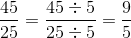That is, 9 to 5 odds against.

### Example Question #16 : How To Find The Probability Of An Outcome

A box contains ten red marbles and fifteen blue marbles. Fifteen more red marbles are added to the box. By how much did the probability that a randomly drawn marble is red increase?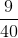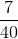Explanation:

Originally, the box contained twenty-five marbles, ten of which were red, so the probability of drawing a red marble was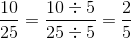After adding fifteen red marbles, the box contains forty marbles, twenty-five of which are red, so the probability of drawing a red marble is now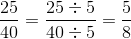The increase in probability is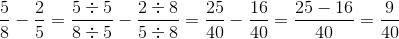### Example Question #17 : How To Find The Probability Of An Outcome

The nines are removed from a standard deck of fifty-two cards. By how much did the probability that a randomly-drawn card will be a heart increase or decrease?

The probability increased by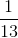.

The probability decreased by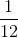.

The probability decreased by.

The probability did not change.

The probability increased by.

The probability did not change.

Explanation:

Before removing the twos, there were fifty-two cards, thirteen of which are hearts, making the probability that a randomly-drawn card would be a heart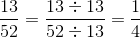After removing the twos, there are forty-eight cards, twelve of which are hearts (one heart, the three of hearts, was removed), making the probability that a randomly-drawn card will be a heart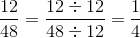The probability did not change.

### Example Question #18 : How To Find The Probability Of An Outcome

A standard deck of fifty-two cards has all of its hearts removed. A card is then drawn at random. Which is the greater quantity?

(A) The probability that the card is a club.

(B) The probability that the card is a 2, a 3, or a 4.

(B) is greater

(A) and (B) are equal

(A) is greater

It is impossible to determine which is greater from the information given

(A) is greater

Explanation:

We need only compare numbers of cards.

Since no clubs were removed, there are thirteen clubs in the modified deck.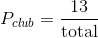Since one 2, one 3, and one 4 were removed, there are three of each, or nine of these cards total, in the modified deck.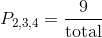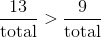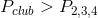The probability of drawing a club, (A), is greater.

### Example Question #19 : How To Find The Probability Of An Outcome

A standard deck of fifty-two cards has all of its aces removed. A card is then drawn at random. Which is the greater quantity?

(A) The probability that the card is a diamond.

(B) The probability that the card is a jack, a queen, or a king.

It is impossible to determine which is greater from the information given

(A) and (B) are equal

(B) is greater

(A) is greater

(A) and (B) are equal

Explanation:

We need only compare numbers of cards.

Since one diamond (the ace of diamonds) was removed, there are twelve diamonds in the modified deck.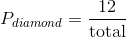Since no jacks, queens, or kings were removed, all four of each (twelve cards total) remain.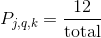This makes the two quantities and, subsequently, the two probabilities, equal.

### Example Question #20 : How To Find The Probability Of An Outcome

Two boxes contain thirty marbles each: red, blue, and green. Box #1 contains ten green marbles and fifteen red marbles; Box #2 contains sixteen green marbles and nine red marbles. Which is the greater quantity?

(A) The probability that a marble randomly drawn from Box #1 is blue.

(A) The probability that a marble randomly drawn from Box #2 is blue.

(A) is greater

(B) is greater

(A) and (B) are equal

It is impossible to determine which is greater from the information given

(A) and (B) are equal

Explanation:

Box #1 contains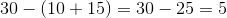blue marbles out of 30 total.

Box #2 contains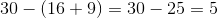blue marbles out of 30 total.

The probability of drawing a blue marble out of Box #1 is the same as that of drawing a blue marble out of Box #2: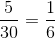.

### All ISEE Middle Level Quantitative Resources